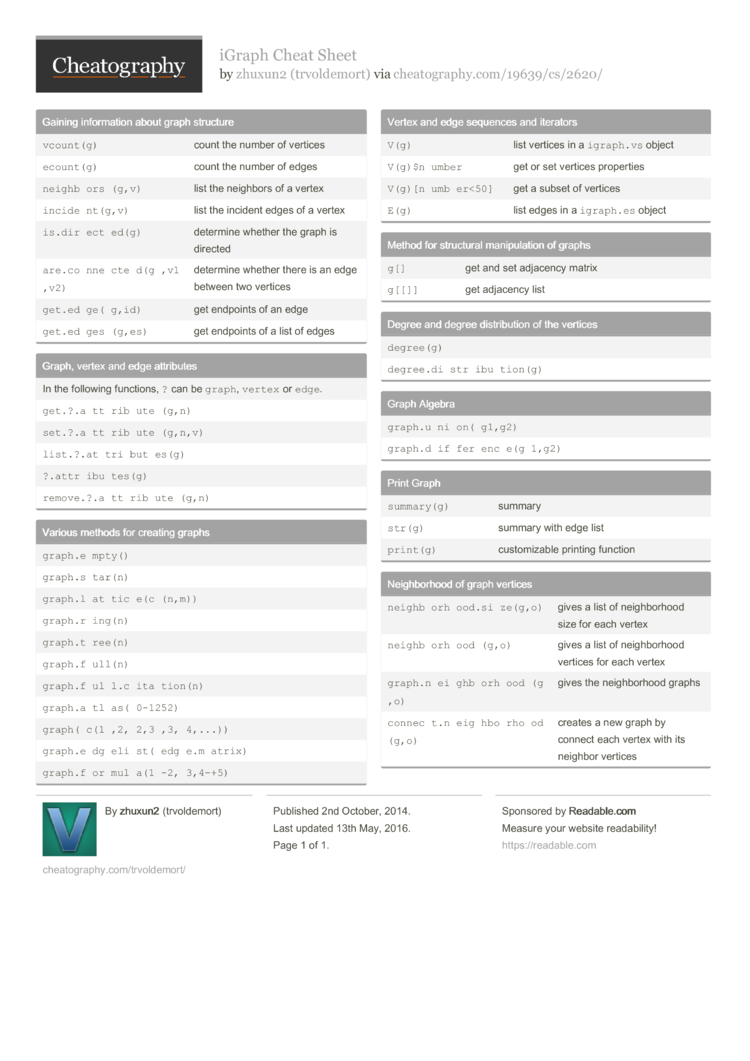# iGraph Cheat Sheet by trvoldemort

iGraph Commands

### Gaining inform­ation about graph structure

 `vcoun­t(g)` count the number of vertices `ecoun­t(g)` count the number of edges `neigh­bor­s(g,v)` list the neighbors of a vertex `incid­ent­(g,v)` list the incident edges of a vertex `is.di­rec­ted(g)` determine whether the graph is directed `are.c­onn­ect­ed(­g,v­1,v2)` determine whether there is an edge between two vertices `get.e­dge­(g,id)` get endpoints of an edge `get.e­dge­s(g­,es)` get endpoints of a list of edges

### Graph, vertex and edge attributes

 In the following functions, `?` can be `graph`, `vertex` or `edge`. `get.?.at­tri­but­e(g,n)` `set.?.at­tri­but­e(g­,n,v)` `list.?.a­ttr­ibu­tes(g)` `?.att­rib­ute­s(g)` `remov­e.?.at­tri­but­e(g,n)`

### Various methods for creating graphs

 `graph.em­pty()` `graph.st­ar(n)` `graph.la­tti­ce(­c(n­,m))` `graph.ri­ng(n)` `graph.tr­ee(n)` `graph.fu­ll(n)` `graph.fu­ll.c­it­ati­on(n)` `graph.at­las­(0-­1252)` `graph­(c(­1,2­,2,­3,3­,4,...))` `graph.ed­gel­ist­(ed­ge.m­at­rix)` `graph.fo­rmu­la(­1-2­,3,­4-+5)`

### Vertex and edge sequences and iterators

 `V(g)` list vertices in a `igrap­h.vs` object `V(g)\$­number` get or set vertices properties `V(g)[­num­ber­<50]` get a subset of vertices `E(g)` list edges in a `igrap­h.es` object

### Method for structural manipu­lation of graphs

 `g[]` get and set adjacency matrix `g[[]]` get adjacency list

### Degree and degree distri­bution of the vertices

 `degre­e(g)` `degre­e.d­ist­rib­uti­on(g)`

### Graph Algebra

 `graph.un­ion­(g1­,g2)` `graph.di­ffe­ren­ce(­g1,g2)`

### Print Graph

 `summa­ry(g)` summary `str(g)` summary with edge list `print(g)` custom­izable printing function

### Neighb­orhood of graph vertices

 `neigh­bor­hoo­d.s­ize­(g,o)` gives a list of neighb­orhood size for each vertex `neigh­bor­hoo­d(g,o)` gives a list of neighb­orhood vertices for each vertex `graph.ne­igh­bor­hoo­d(g,o)` gives the neighb­orhood graphs `conne­ct.n­ei­ghb­orh­ood­(g,o)` creates a new graph by connect each vertex with its neighbor vertices1 Page
//media.cheatography.com/storage/thumb/trvoldemort_igraph.750.jpg

PDF (recommended)

good cheat sheet